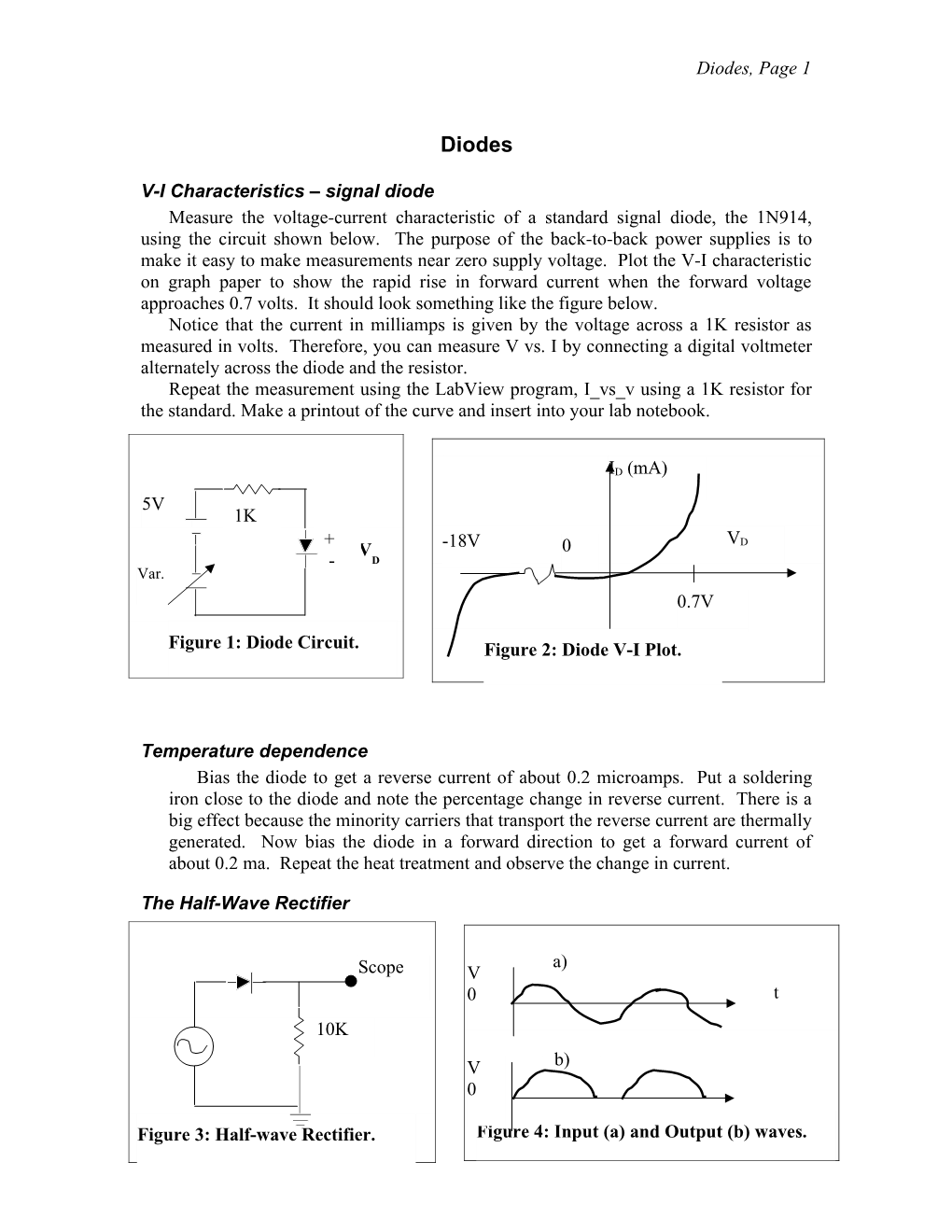# V-I Characteristics Signal DiodeDiodes, Page 1

## Diodes

### V-I Characteristics – signal diode

Measure the voltage-current characteristic of a standard signal diode, the 1N914, using the circuit shown below. The purpose of the back-to-back power supplies is to make it easy to make measurements near zero supply voltage. Plot the V-I characteristic on graph paper to show the rapid rise in forward current when the forward voltage approaches 0.7 volts. It should look something like the figure below.

Notice that the current in milliamps is given by the voltage across a 1K resistor as measured in volts. Therefore, you can measure V vs. I by connecting a digital voltmeter alternately across the diode and the resistor.

Repeat the measurement using the LabView program, I_vs_v using a 1K resistor for the standard. Make a printout of the curve and insert into your lab notebook.

### Temperature dependence

Bias the diode to get a reverse current of about 0.2 microamps. Put a soldering iron close to the diode and note the percentage change in reverse current. There is a big effect because the minority carriers that transport the reverse current are thermally generated. Now bias the diode in a forward direction to get a forward current of about 0.2 ma. Repeat the heat treatment and observe the change in current.

### The Half-Wave Rectifier

The basic half-wave rectifier with resistive load looks like the circuit above. The Input and Output waveforms are shown on the right.

The half-wave rectifier with filter capacitor and resistive load is shown in Figure 6 with the input and output waveforms in Figure 5.

The capacitor is electrolytic. You must obey the polarity markings when you connect this capacitor in the circuit.

Use an 8 VRMS 60-Hz transformer secondary as a source.

(a)Begin with the capacitor disconnected. Observe the half-wave rectified pattern on the oscilloscope. Verify that the frequency of the pattern is 60 Hz.

(b)Add the 10-F capacitor, getting the polarity right, but remove the load resistor. Observe how the capacitor integrates the rectified power to make a smooth DC source. We might imagine using such a source to power some electronics that need a stable source.

(c)Add the resistive load back into the circuit and see how the load causes the output of the power supply to “ripple”. You may need to AC couple the scope and increase the gain to see the ripple well. Verify that the frequency of the ripple is 60 Hz. Use the dual trace feature of the scope to find the phase of the ripple with respect to the phase of the input AC voltage. On what phase of the AC input does the capacitor charge?

### The Full-Wave Rectifier

The full-wave rectifier, with filter capacitor and resistive load, is shown in Figure 8 with the input and output waveforms in Figure 7.

Build the circuit above. Use an 8-VRMS, 60-Hz supply.

(a)Begin with the capacitor disconnected. Observe the full-wave rectified pattern on the oscilloscope. Verify that the frequency of the pattern is 120 Hz. [Note: Because the two channels of your oscilloscope have a common ground, it is not possible to view both the input and the output of the rectifier at once.]

(b)Add the 10-F capacitor, getting the polarity right, but remove the load resistor. Observe how the capacitor integrates the rectified power to make a smooth DC source.

(c)Add the resistive load back into the circuit and see how the load causes the output of the power supply to “ripple.” Verify that the frequency of the ripple is 120 Hz.

(d)Reduce the resistive load by making the resistor 5 K instead of 1 K. Observe that the ripple decreases.

Explain the advantage of the full-wave over the half-wave rectifier in making a stable power supply. In fact, neither supply is very good. Both are unregulated. Regulated power supplies will be studied in a later lab.

### The Zener Diode

Use the LabView program to measure the V-I characteristic for a Zener diode, the 1N4734, which has a Zener voltage of 5.6 v. (Note: In the forward condition, current flows out of the terminal labeled with the band.) Enter the resulting plot in your notebook. A Zener diode, biased at this breakdown condition, is a stable voltage reference because the voltage does not change much even though the current may change.

### The PIN Diode

The PIN diode has intrinsic (not doped) material (I) in the junction between P and N type material. It makes a fast photo diode, wherein minority carriers are generated by photons. Because the photoeffect depends upon minority carriers, the diode is biased in the reversed condition for normal operation as a light-sensitive device. Use the PN323B pin diode in the circuit in Fig. 9 to show a large increase in diode current when a bright lamp is brought close to the diode. What is the effect of elevated temperature on the reverse current?

Reverse the diode to attempt to find the effect of light on the diode current in the forward-biased condition.

### The interrupter

The interrupter consists of an LED and a phototransistor separated by a gap (see Figure 10). When the gap is empty the light (infrared) from the LED excites carriers in the phototransistor, which then conducts. When the gap is filled, the light is interrupted and the phototransistor does not conduct much.

Build the circuit in Figure 11 using an H22A1 from Harris Semiconductor.

Can you interrupt the light path so fast that you test the speed of the response? Better be speedy. The turn-on time is 8s. The turn-off time is 50s.

Interrupters are used with spinning disks with holes that allow the light to pass. A simple counter on the output of the phototransistor can count the number of revolutions, and with the addition of a timing circuit the speed of the disk can be determined.